### Home > CC1MN > Chapter 6 > Lesson 6.1.1 > Problem6-4

6-4.

The diagrams below show the portion of another class mural that Josephine was supposed to paint and how much she actually did paint. Use the pictures to answer the questions that follow.

The portion Josephine was supposed to paint: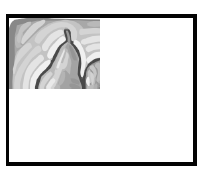The portion Josephine actually painted: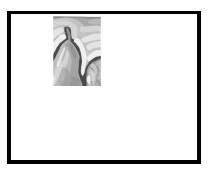1. Approximately what portion of the painting was Josephine supposed to paint?

• Lines have been drawn on the first mural to help you estimate.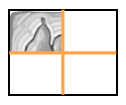1. Approximately what fraction of her assigned portion did Josephine actually complete?

• Another line has been drawn on the second mural to help you estimate.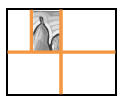1. Write a product to show what portion of the mural Josephine actually painted.

• A few more lines have been added to the diagram.

• $\text{How can you write }\frac{1}{2}\text{ of }\frac{1}{4} \text{ as a product?}$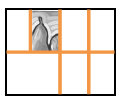$\left ( \frac{1}{2} \right )\left ( \frac{1}{4} \right )=\frac{1}{8}$